# Similar Polygons: Ratio of Areas, Perimeters, and Side Lengths

• Author:
• Updated date:

Ray is a licensed engineer in the Philippines. He loves to write about mathematics and civil engineering.

Similar polygons are polygons whose corresponding angles are congruent, and whose lengths of corresponding sides are proportional. The idea of similarity of triangles can easily be extended to that of n-sided polygons, for n > 3. Similar polygons are polygons whose ratio of the perimeters is equal to the scale factors of the two polygons. Scale factors are the common ratio of the lengths of two corresponding sides of the two similar polygons. They can be expressed as fractions or decimals.

Scale Factor = Perimeter of larger polygon / Perimeter of smaller polygon

Scale Factor = Longer length of polygon 1 / Shorter corresponding length of polygon 2

In similar triangles, the ratio of the areas is equal to the square of the ratio of the corresponding sides. The same goes with two similar polygons for n > 3. The area ratio of two similar polygons is equal to the square of the proportion of any two corresponding sides and two corresponding diagonals.

AL / As = (SL/SS)2

AL / As = (dL/dS)2

Where:

AL = area of the larger polygon

As = area of the smaller polygon

SL = length of the longer corresponding side

SS = length of the shorter corresponding side

dL = length of the longer corresponding diagonal

dS = length of the shorter corresponding diagonal

In the figures shown, assume that hexagon ABCDEF and PQRSTU are similar. From the definition of similar polygons, we can say that ∠A ≅ ∠P, ∠B ≅ ∠Q, ∠C ≅ ∠R, ∠D ≅ ∠S, ∠E ≅ ∠T, and ∠F ≅ ∠U.

Scroll to Continue

For instance, consider the following quadrilaterals given their sides. Note that ∠A ≅ ∠P, ∠B ≅ ∠Q, ∠C ≅ ∠R, ∠D ≅ ∠S. Finding the ratios of the corresponding sides, we get the following.

The given solution above implies that AB/PQ = BC/QR = CD/RS = DA/SP = 5. By the definition of similar polygons, we can infer that quadrilaterals ABCD and PQRS are similar. In symbols, □ ABCD ~ □ PQRS.

As you can observe, the common ratio 5 is the scale factor of □ ABCD to □ PQRS. It means that all side lengths of □ PQRS shall be multiplied with the scale factor 5 to obtain the dimension of the sides of □ ABCD since ABCD is the larger quadrilateral.

## How to Know If Two Polygons Are Similar

To guarantee that the n-sided polygons, n > 3, are similar, it is necessary that both conditions in the definition of similar polygons are satisfied. Even if one knows that the corresponding angles of two polygons are congruent, it does not follow that the polygons are similar. So, for us to verify if two polygons are similar, two conditions must be satisfied.

1. The corresponding angles of the two polygons are congruent.
2. The lengths of corresponding sides are proportional.

Observe the given figure A. Notice that the corresponding angles are all right angles, and thus, are congruent, but the corresponding sides are not proportional. Also, the proportionality of corresponding sides of two polygons does not guarantee that the polygons are similar. Also, take a look at figure B. The square PQRS and rhombus XYZW have proportional sides but are not identical.

## Example 1: Finding the Lengths and Perimeters Given the Scale Factor

The quadrilaterals ABCD and EFGH are similar with a scale factor ⅓.

1. Find the lengths a, b, c, d of quadrilateral EFGH.
2. Find the ratio of the perimeters of quadrilateral ABCD and EFGH.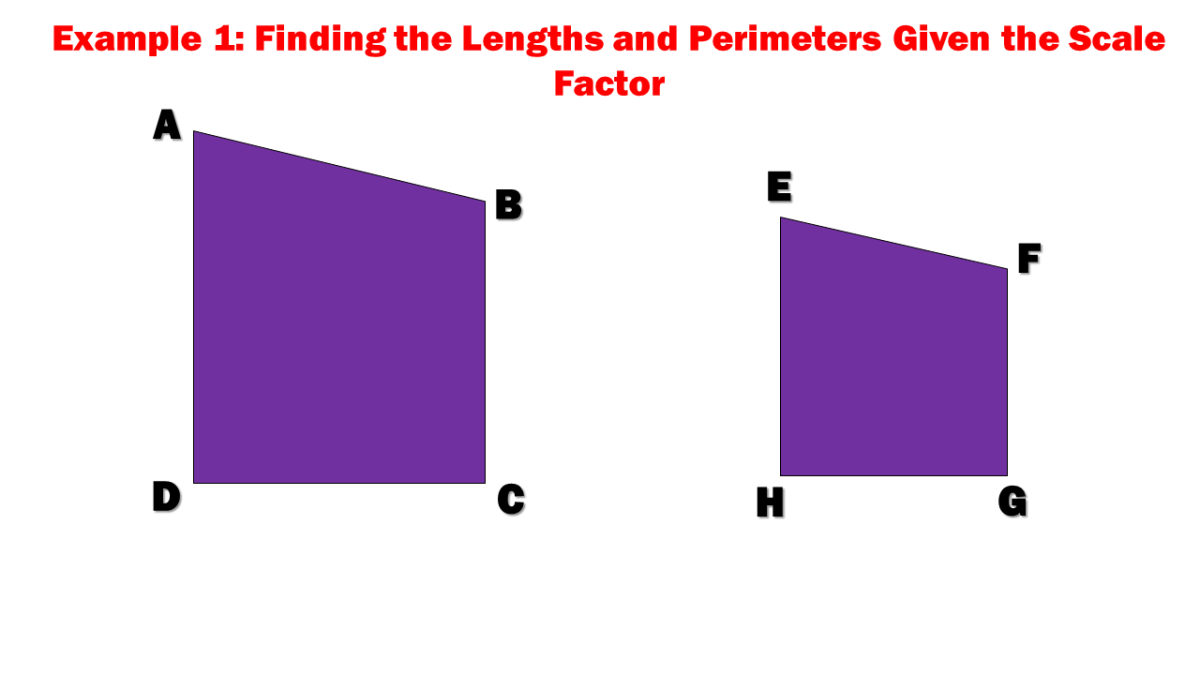Example 1: Finding the Lengths and Perimeters Given the Scale Factor

Solution

Given the scale factor of ⅓, compute for the values of a, b, c, and d of quadrilateral EFGH by multiplying the given side lengths of ABCD with the scale factor. Take note that the scale factor is equal to the longer length divided by, the shorter length.

Scale factor = larger length / shorter length

⅓ = a / EF

⅓ = a / 5

a = 5 / (⅓)

a = 5 x 3

a = 15

⅓ = b / BC

⅓ = b / 2

b = 2 / (⅓)

b = 2 x 3

b = 6

⅓ = c / DC

⅓ = c / 3

c = 3 / (⅓)

c = 3 x 3

c = 9

⅓ = d / 4

d = 4 / (⅓)

d = 4 x 3

d = 12

For the ratio of the perimeters of ABCD and EFGH, compute for the value of the edges.

P (ABCD) = 2 + 3 + 4 + 5

P (ABCD) = 14

P (EFGH) = 6 + 9 + 12 + 15

P (EFGH) = 42

Ratio of Perimeters = Smaller Perimeter / Larger Perimeter

Ratio of Perimeters = 14 / 42

Ratio of Perimeters = ⅓

As you can observe, the ratio of the perimeter is equal to the given scale factor.

The lengths a, b, c, d of quadrilateral EFGH are 15, 6, 9, and 12, respectively. The ratio of the perimeters of ABCD and EFGH is ⅓ which is equal to the given scale factor.

## Example 2: Finding the Length of Corresponding Sides of Similar Polygons

The area of two similar polygons is 120 cm2 and 30 cm2. If a side of the smaller polygon has a length of 3 centimeters, find the length of the corresponding side of the larger polygon.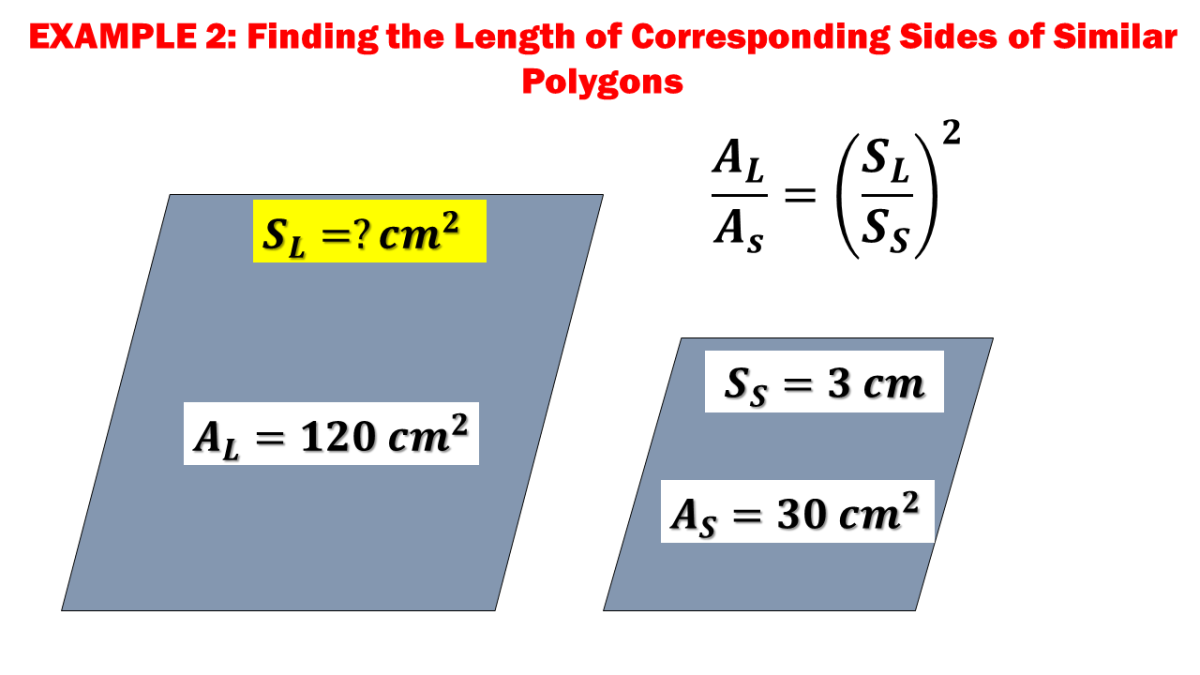Example 2: Finding the Length of Corresponding Sides of Similar Polygons

Solution

Let AL be the area of the larger polygon and AS be the area of the smaller polygon. Take note that the given length of the side of the smaller polygon SS is 3 centimeters. Substitute the given quantities to the equation.

AL = 120 cm2

AS = 30 cm2

SS = 3 cm

AL / AS = (SL/SS)2

120 / 30 = (SL/3)2

4 = (SL/3)2

4 = SL2 / 9

SL2 = 9 x 4

SL2 = 36

SL2 = √36

SL = 6 cm

The length of the corresponding side of the larger polygon SL is equal to 6 centimeters.

## Example 3: Finding the Area of the Smaller Polygon Given the Diagonals and Areas

The corresponding diagonals of two similar polygons have lengths 4 cm and 7 cm. If the area of the larger polygon is 147 square centimeters, find the value of As.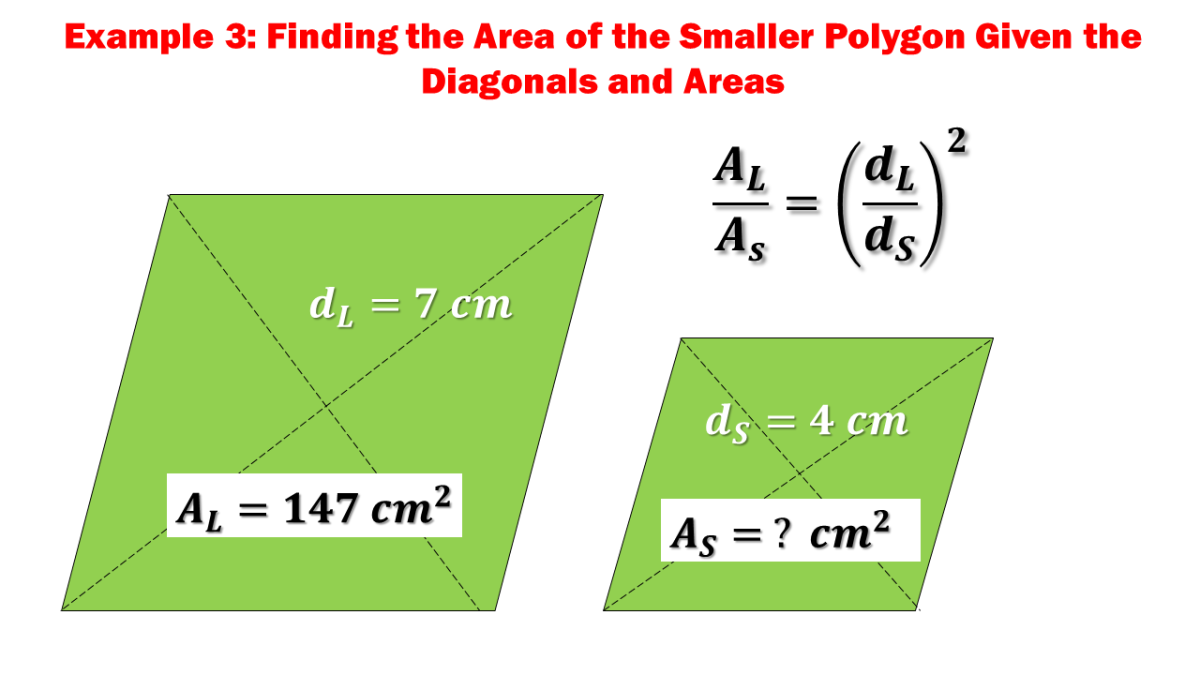Example 3: Finding the Area of the Smaller Polygon Given the Diagonals and Areas

Solution

Let dL be the length of the diagonal of the larger polygon, and dS that of the corresponding diagonal of the smaller polygon. Given that AL = 147 cm2, compute the smaller polygon's area using the mentioned formulas earlier.

AL = 147 cm2

ds = 4 cm

dL = 7 cm

AL / AS = (dL/dS)2

147 / AS = (7/4)2

147 / AS = 49/16

AS = 147 (16) / (49)

AS = 48 cm2

The area of the smaller polygon is equal to As = 48 cm2.

## Example 4: Finding the Scale Factors, Side Lengths, and Perimeters of Similar Polygons

Given that □PQRS ~ □JKLM, find the following.

1. Scale factor of PQRS to JKLM
2. Scale factor of JKLM to PQRS
3. Values of x,y, and w
4. The perimeter of each polygon
5. The ratio of the sum of edges of □PQRS to the sum of sides of □JKLM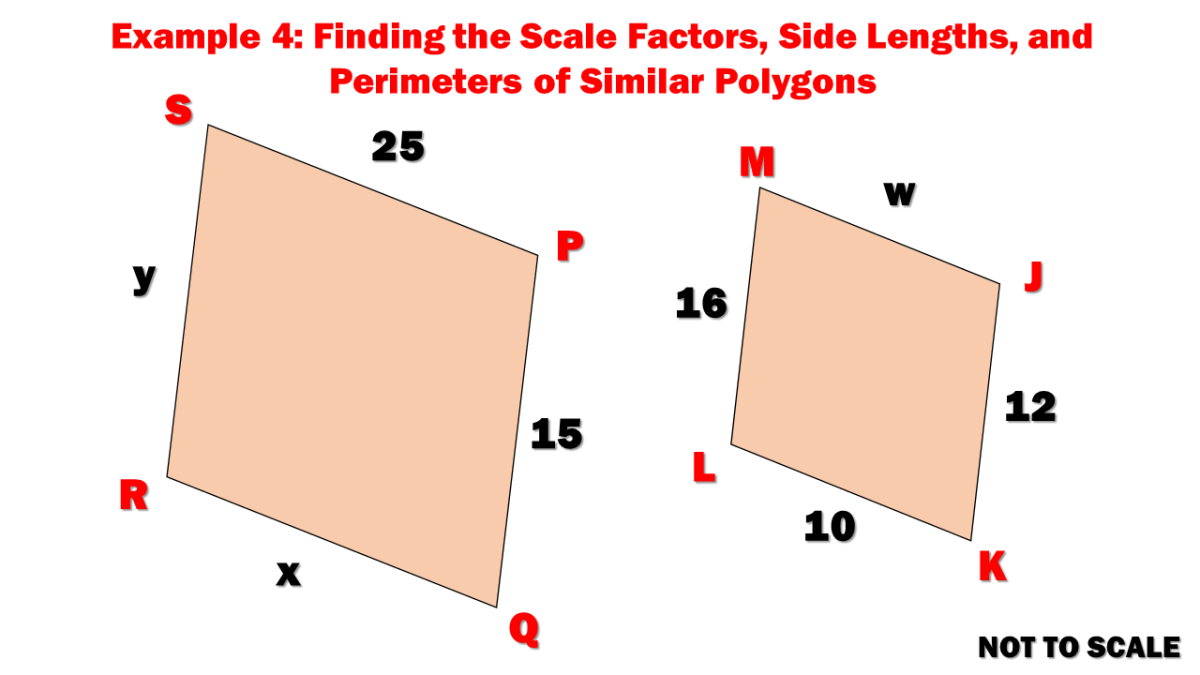Example 4: Finding the Scale Factors, Side Lengths, and Perimeters of Similar Polygons

Solution

In obtaining the scale factor of PQRS to JKLM, try to look for side lengths given in the figure. Divide the given length of PQRS by the corresponding side length of JKLM. On the other hand, solve for the scale factor of JKLM to PQRS by reversing the mentioned procedure by dividing the size of the corresponding sides of JKLM by that of PQRS.

PQ / JK = 15 / 12

PQ / JK = 4/3

JK / PQ = 12 / 15

JK / PQ = ¾

Since we have obtained the scale factors of JKLM to PQRS and PQRS to JKLM, we may now compute for the values of x, y, and w.

x = KL x (4/3)

x = 10 x (4/3)

x = 40/3 units

y = LM x (4/3)

y = 16 x (4/3)

y = 64/3 units

w = PS x (¾)

w = 25 x (¾)

w = 75/4 units

Now that all side lengths of similar polygons are complete obtain the perimeter by simply adding all the sides separately. Then, get the ratio of the perimeter of □ PQRS to the perimeter of □JKLM.

P (PQRS) = 25 + 15 + 40/3 + 64/3

P (PQRS) = 224/3 = 74.6 units

P (JKLM) = 12 + 10 + 16 + 75/4

P (JKLM) = 227/4 = 56.75 units

The ratio of perimeters = Perimeter of PQRS / Perimeter of JKLM

The ratio of perimeters = 74.6 / 56.75

The ratio of perimeters = 4/3

The scale factors of PQRS to JKLM and JKLM to PQRS are 4/3 and ¾, respectively.

The values of x, y, and w are 40/3, 64/3, and 75/4, respectively.

The perimeter of PQRS is 74.6 units.

The perimeter of JKLM is 56.75 units.

The perimeter ratio of PQRS to JKLM is 4/3, which is equal to the scale factor of PQRS to JKLM.

## Example 5: Finding the Scale Factor Given the Perimeters of Similar Parallelograms

The perimeter of parallelograms PQRS and JKLM are 94 cm and 18.8 cm, respectively. If the two parallelograms are similar, find the scale factor of parallelogram PQRS to parallelogram JKLM.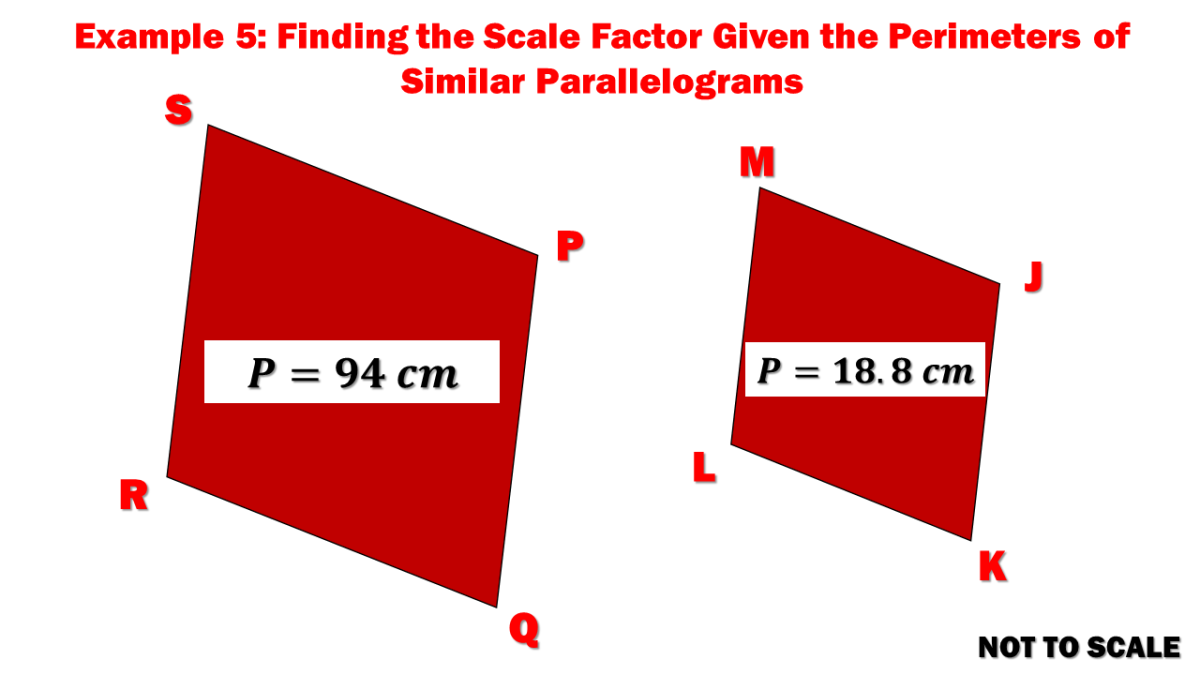Example 5: Finding the Scale Factor Given the Perimeters of Similar Parallelograms

Solution

Remember that the ratio of perimeters of two similar polygons is equal to their scale factor. To obtain the scale factor of parallelogram PQRS to JKLM, divide the perimeter of PQRS, which is 94 centimeters, to the circumference of JKLM, which is 18.8 centimeters.

Scale Factor of PQRS to JKLM = P (PQRS) / P (JKLM)

Scale Factor of PQRS to JKLM = 94 / 18.8

Scale Factor of PQRS to JKLM = 5

The scale factor of parallelogram PQRS to parallelogram JKLM is equal to five.

## Example 6: Solving Similar Polygons Problem Given the Ratio of Areas

The areas of two similar triangles are in the ratio 25:16.

1. Find the length of a side larger triangle if the corresponding side of the smaller triangle has a length of 80 units.
2. Find the length of the medians of the larger triangle if the corresponding median of the smaller triangle has a length of 10 units.
3. Find the length of an angle bisector of the smaller triangle if the corresponding angle bisector of the larger triangle has a length of 15 units.
4. Find the perimeter of the smaller triangle if the perimeter of the larger triangle is 125.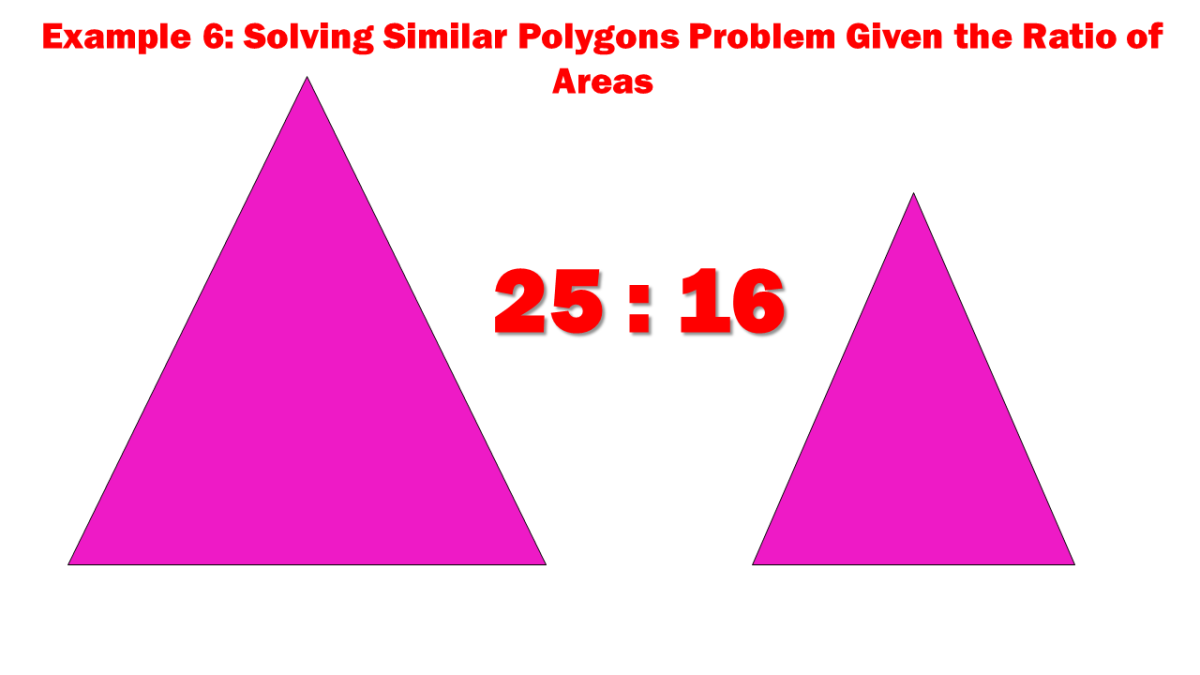Example 6: Solving Similar Polygons Problem Given the Ratio of Areas

Solution

We are given the ratio of areas of two similar triangles, which is 25:16. In finding the length of a side of the larger triangle given the corresponding side of the smaller triangle SS = 80 units, use the formula for the ratio of areas and corresponding sides.

AL / AS = (SL/SS)2

25 / 16 = (SL/80)2

SL = 100 units

For the questions relating to medians and angle bisectors, remember that two triangles are similar if the ratio of any two corresponding segments (altitudes, medians, or angle bisectors) is equal to the proportion of any two corresponding sides. Now that we have obtained the value of the shorter side create a proportion formula for the side lengths and corresponding segments such as medians and angle bisectors.

SL / SS = ML / MS

100 / 80 = ML / 10

ML = 12.5 units

SL / SS = ABL / ABS

100 / 80 = 15 / ABS

ABS = 12 units

Finally, solve for the perimeter of the smaller triangle given the perimeter of the larger triangle PL = 125. Recall that the ratio of perimeters of two similar polygons is equal to the ratio of the lengths of two corresponding sides.

PL / PS = SL / SS

125 / PS = 100 / 80

PS = 100 units

The length of the side of the larger triangle is equal to 100 units.

The length of the medians of the larger triangle is equal to 12.5 units.

The length of the angle bisector of the smaller triangle is equal to 12 units.

The perimeter of the smaller triangle is equal to 100 units.

## Example 7: Solving the Area of the Larger Triangle Given the Perimeters of Similar Polygons

Two similar polygons have perimeters of 32 cm and 24 cm. If the area of the smaller triangle is 27 square centimeters, find the area of the larger triangle.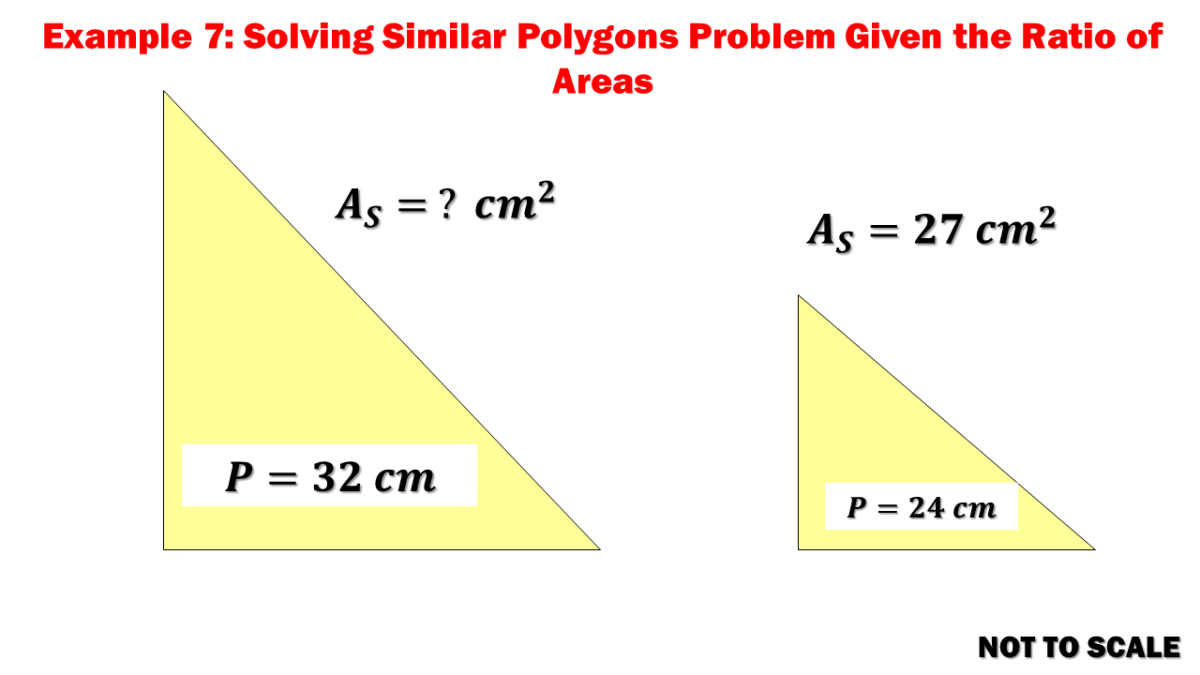Example 7: Solving the Area of the Larger Triangle Given the Perimeters of Similar Polygons

Solution

Given the perimeters of the two similar polygons, compute for the ratio of the corresponding sides. Once the ratio of the corresponding sides is available, compute the area of the smaller triangle by using the formula for the ratio of areas and corresponding sides.

PL / PS = SL / SS

32 / 24 = SL / SS

SL / SS = 4/3

AL / As = (SL/SS)2

AL / 27 = (4/3)2

AL = 48 square centimeters

The area of the larger triangle is equal to 48 square centimeters.

## Example 8: Similar Polygons Given the Sum of the Areas and Perimeters

The sum of the areas of the two similar polygons is 65 square units. If their perimeters are 12 units and 18 units, find the area of a larger polygon.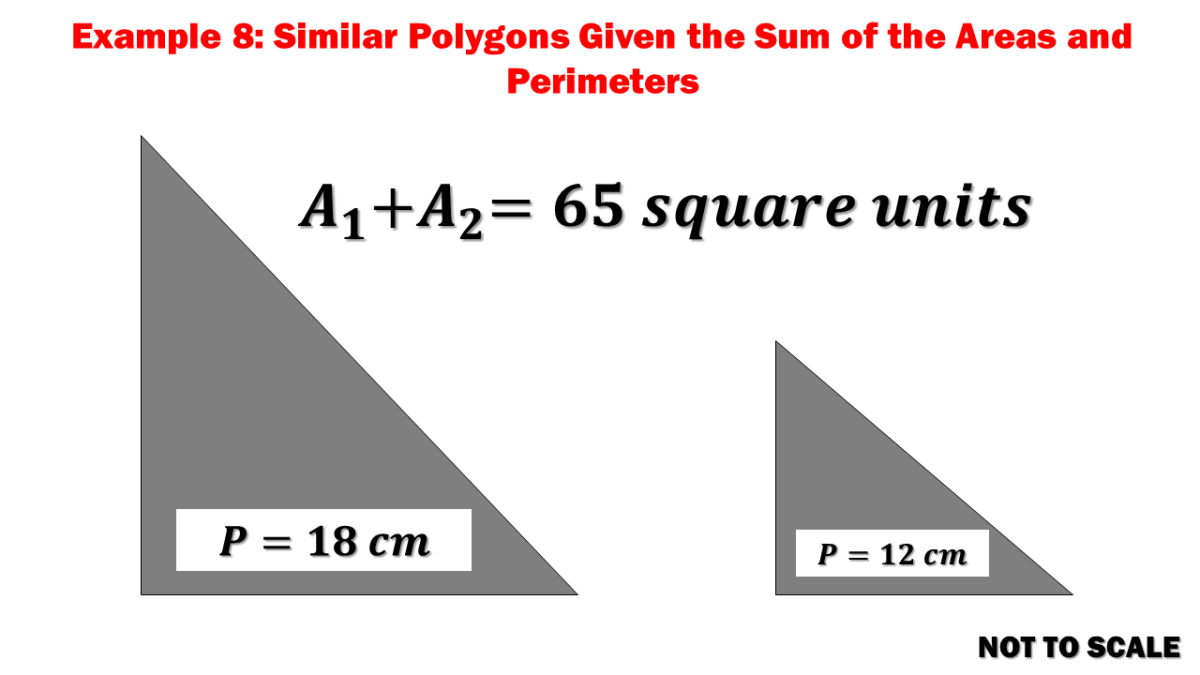Example 8: Similar Polygons Given the Sum of the Areas and Perimeters

Solution

Create a mathematical equation describing the sum of the areas of two similar polygons. From there, express the area of the smaller polygon in terms of the larger polygon.

AL + AS = 65 square units

AS = 65 - AL

Compute the proportion of the corresponding lengths of the two similar polygons by utilizing the given perimeters.

PL = 18 units

PS = 12 units

PL / PS = SL / SS

18 / 12 = SL / SS

SL / SS = 3/2

Use the formula for the ratio of areas and corresponding sides. Substitute the arrived equation for the area of the smaller polygon to the equation.

AL / AS = (SL/SS)2

AL / (65 - AL) = (3/2)2

AL = 45 square units

The area of a larger polygon is 45 square units.

## Example 9: SImilar Polygons Word Problem

Suppose Alfred wants a wallet size photo to be similar to an 8 x 10 photo. If the wallet size photo were 2.5 inches wide, how long would it be?

Solution

The larger polygon is of the size 8 inches x 10 inches based on the given wallet-size photo. Recall that similar polygons are similar if the lengths of their corresponding sides are proportional. Compute for the height of the wallet size photo by creating a proportion equation.

8 / 2.5 = 10 / y

y = 3.125 inches

The wallet size photo dimensions are 2.5 inches and 3.125 inches.

## Example 10: Scale Factors and Side Lengths of Similar Polygons

Identify the dilation, and find its scale factor. Then, find the values of x, y, and z.

Solution

Dilation is a condition of becoming or being made expanded, more extensive, or more open. Therefore, polygons dilated can be inferred as similar polygons. Compute the scale factor of the polygon and the dilated one by getting the ratio of the two corresponding sides. Once computed, compute for the values of x, y, and z by finding the ratios of the corresponding sides.

Scale Factor = P’Q’ / PQ

Scale Factor = 10 / 20

Scale Factor = ½

Scale Factor = 8 / x

½ = 8 / x

x = 16 units

Scale Factor = 10 / y

½ = 10 / y

y = 20 units

Scale Factor = 8 / z

½ = 8 / z

z = 16 units